Writing Subtraction Equations

How do your kid's subtraction skills stack up? His academic confidence will soar to new heights after practising some two-digit subtraction!

Click on a worksheet in the set below to see more info or download the PDF.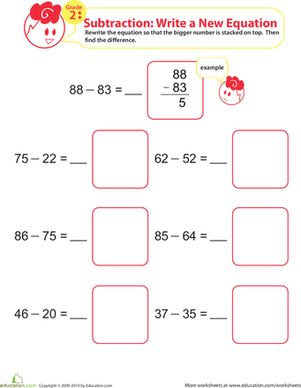Subtraction Without Regrouping

This printable maths worksheet asks your second grader to rewrite each subtraction equation, stacking the larger number on top, and write down each answer.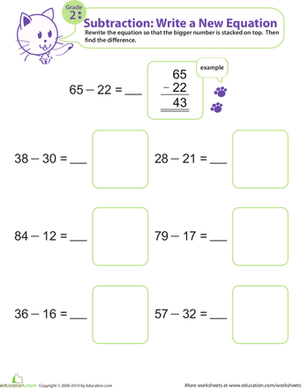Writing Subtraction Equations 2

Provide your second grader with a healthy subtraction boost with this kitten-adorned worksheet. She will be practising subtraction in no time!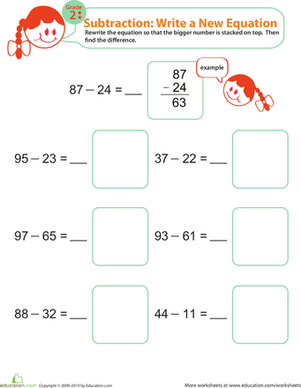Writing Subtraction Equations 3

Help your second grader regain her mathematical confidence by providing her with this two-digit subtraction worksheet.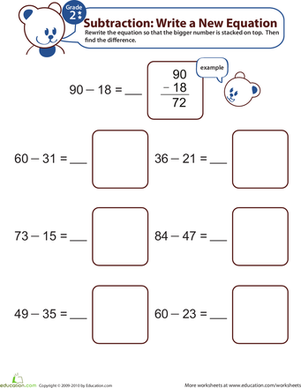Writing Subtraction Equations 4

This printable page of subtraction problems will give your second grader invaluable two-digit subtraction practise and a familiarity with writing equations.Writing Subtraction Equations 5

Your child will rewrite each equation according to the directions, then find the answers. Your child will practise solving problems and writing equations too!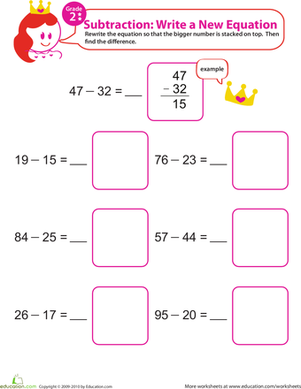Writing Subtraction Equations 6

Help your child become a subtraction master with this subtraction worksheet. Your child will rewrite the subtraction equation, then find the difference.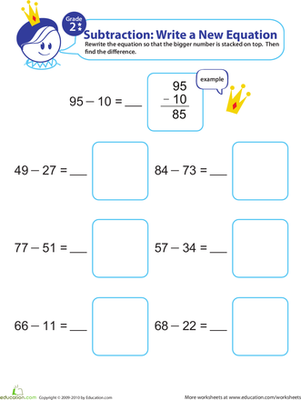Writing Subtraction Equations 7

Help your kid perfect his subtraction skills with this maths worksheet. Your kid will get subtraction practise and learn to express equations in different ways.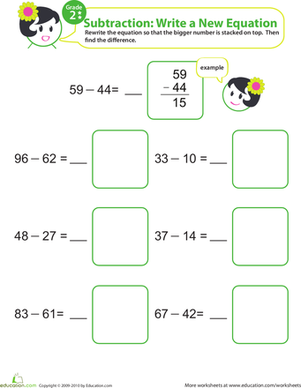Writing Subtraction Equations 8

Two-digit subtraction can be the bane of a second grader's existence. Encourage your child to do some extra practise at home with this adorable printable.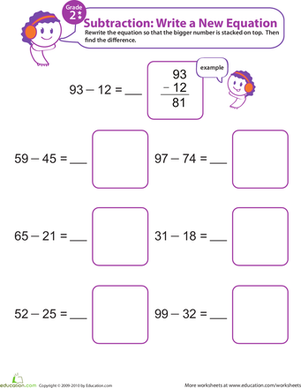Writing Subtraction Equations 9

Give your kid a boost in maths with this fun subtraction worksheet. For each problem, your kid will rewrite the equation a different way and find the difference.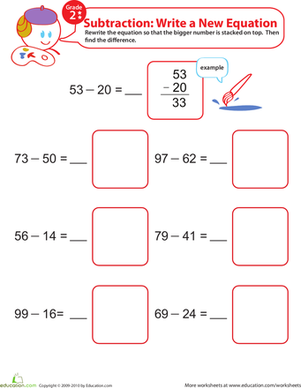Writing Subtraction Equations 10

Mr. Artist needs to solve a set of subtraction problems, but he has trouble understanding these equations as they are written. Will your child give him a hand?

Want to download this whole set as a single PDF?

Create new collection

0

New Collection>

0Items

What could we do to improve Education.com?

Please note: Use the Contact Us link at the bottom of our website for account-specific questions or issues.

What would make you love Education.com?